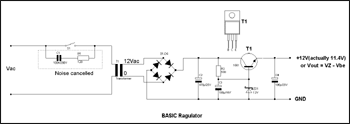# Basic Regulated Power Supply

This is a basic regulated circuit power supply.The heart of this circuit is ZD1 on the base of transisitor T1 which is determine the require output voltage.So if you need to change the output voltage you just change the zener diode rate voltage.

Remeber that the output voltage alway is less than base pin voltage 0.6V for example if we use 12V zener diode thus the output voltage is about 11.4V .

The maximum output current is determined by hfe of transistor T1 multiplied by base current(Ib) which determined by R2.

Note the rated voltage of capacitor C4 must be greater than the output volatge.The R1 and C1 is noise cancelled when the main power switch turn on to reduce noise into our circuit.